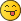#8
(2019-10-30, 2:48)N. W. Perry Wrote: There's another way to do this, by finding the intersection of two circles. This might be handy if you need to do it in a program that doesn't have LDCad's triangle calculation feature (or if, as I did, you forgot that feature exists and spent an hour figuring it out for yourself and are feeling all clever about it).I setup the selection info stuff before the scripting existed and didn't want to force a certain way to apply the angles etc.

But you could script your method, and apply the angles in one go if wanted.

FYI: This is what the selection info does internally:

Code:
```        const double a=relPnt.length(relPnt); const double b=relPnt.length(relPnt); const double c=relPnt.length(relPnt); const double angAB=rad2Deg(acos((a*a+b*b-c*c)/(2*a*b))); const double angBC=rad2Deg(acos((b*b+c*c-a*a)/(2*b*c))); TGLVector3d BADir=relPnt-relPnt; BADir.normalize(); TGLVector3d BCDir=relPnt-relPnt; BCDir.normalize(); TGLVector3d CBDir=BCDir*-1.0; TGLVector3d CDDir=relPnt-relPnt; CDDir.normalize(); TGLVector3d planeNor(true); planeNor.comp[sCompIdx]=1.0; const double intAngAB=rad2Deg(planeNor.calcSignedAngleBetween(BCDir, BADir)); const double intAngBC=rad2Deg(planeNor.calcSignedAngleBetween(CBDir, CDDir)); const double angABCor=intAngAB+angAB; const double angBCCor=intAngBC-angBC; const double angABCorInv=intAngAB-angAB; const double angBCCorInv=intAngBC+angBC;```
« Next Oldest | Next Newest »